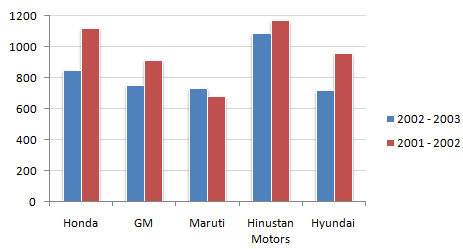# Data Interpretation - Bar Charts - Discussion

Study the following bar chart and answer the questions carefully.

Sales Turnover of 5 Companies (in Rs. crores)5.

What is the approximate difference between the average sales turnover of all the companies put together between the years 2001 - 2002 and 2002 - 2003 ?

 [A]. 133.45 [B]. 142.48 [C]. 117.6 [D]. None of these

Explanation:

Difference between the sum of the two years divided by 5.

 Swojeena Shrestha said: (May 1, 2014) Since there is not given number to calculate exact turnover in number. So, how can we determine answer, even in point?

 Kasinath @Hyd said: (Aug 20, 2014) 2001-02:(1100+900+650+1150+950) = 3750. Avg = 3750/5 2002-03: 3050. Avg = 3050/5. Difference = (3750-3050)/5 = 140.

 Deepu said: (Mar 15, 2020) It must be none of these option D.